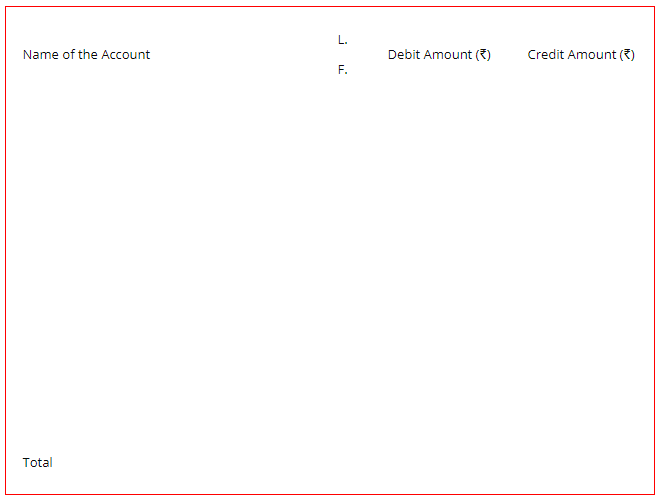# Preparing Trial Balance: Objectives, Trial Balance Format, Examples etc

The compilation of these Trial Balance and Rectification of Accounting Errors Notes makes students exam preparation simpler and organised.

## Meaning and Objectives of Preparing Trial Balance

We all know that we do accounting for financial transactions on the basis of the dual aspect concept of accounting. It means that every debit has a respective credit. Thus, we can say that the sum of all debits is equal to the sum of all credits. For this purpose, we presume that all the entries in the ledger accounts are correct and accurate. Here, we will learn the meaning and objectives of trial balance along with the Trial Balance Format.

### Preparing Trial Balance

A Trial Balance is a statement that shows the total debit and total credit balances of accounts. The total of debit amounts shall be equal to the credit amounts. It thus verifies the arithmetical accuracy of the postings in the ledger accounts. It is a significant statement as it shows the final balances of all the accounts and also aids in the preparation of the Final Accounts.

While preparing the final accounts the balances of accounts are directly taken from the Trial Balance. A firm normally prepares the trial balance at the end of the accounting year. However, it may also prepare it monthly, quarterly or half-yearly also depending upon its requirements.

### Objectives of Preparing Trial Balance

1. Ascertainment of the Arithmetical Accuracy
We record the entire debit as well as the credit balances of the ledger accounts in a Trial Balance. In other words, it is the summary of all the ledger accounts. The total of debit side shall be equal to the credit side, for the trial balance to get tallied. When it tallies, we assume that the posting and the balancing of the ledger accounts are accurate. Thus, it ensures the arithmetical accuracy of the ledgers. However, this is not conclusive evidence of the correctness and the accuracy of the ledger accounts.

2. Locating the Errors
It helps in locating the errors in the posting or recording of the transactions. When a Trial Balance does not tally, then we know that the errors must have occurred at the time of

• totaling the Subsidiary books
• posting in the ledgers
• balancing the accounts
• writing the account balances in the trial balance
• totaling the trial balance. After finding the errors we need to rectify them.

3. Preparation of Financial Statements
The Trial Balance is a summary of all the ledger account balances. Thus, while preparing the final accounts, we do not need to refer to the individual accounts. We can take the final balances of the accounts directly from the Trial Balance. We directly transfer the final balances to the Trading and Profit and Loss A/c and Balance Sheet. Let us now look at the trial balance format.### Trial Balance Format

Trial Balance of……..
As on.……Example:

Question:
Explain the steps in the preparation of the Trial Balance?